# Selina Solutions Concise Mathematics Class 6 Chapter 26: Triangles Exercise 26(B)

Selina Solutions Concise Mathematics Class 6 Chapter 26 Triangles Exercise 26(B) are designed with the aim of improving problem solving abilities among students. Construction of triangles is the main concept talked about under this exercise. The solutions give a clear idea of steps to be followed in construction of triangles. Students can cross check their answers and the method of solving, by using solutions curated by faculty at BYJU’S. Referring solved examples; students acquire better conceptual knowledge, which is important from the exam point of view. Selina Solutions Concise Mathematics Class 6 Chapter 26 Triangles Exercise 26(B), PDF links which are given below for free download.

## Selina Solutions Concise Mathematics Class 6 Chapter 26: Triangles Exercise 26(B) Download PDF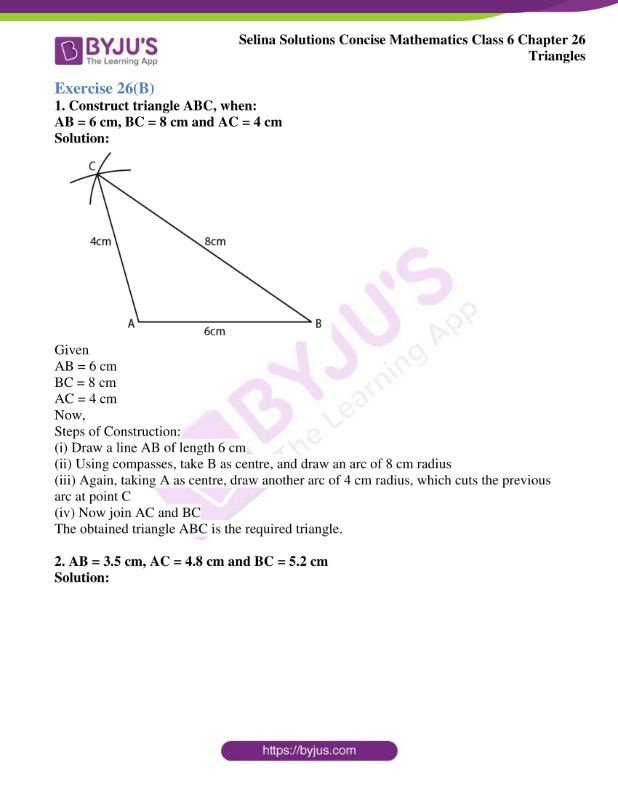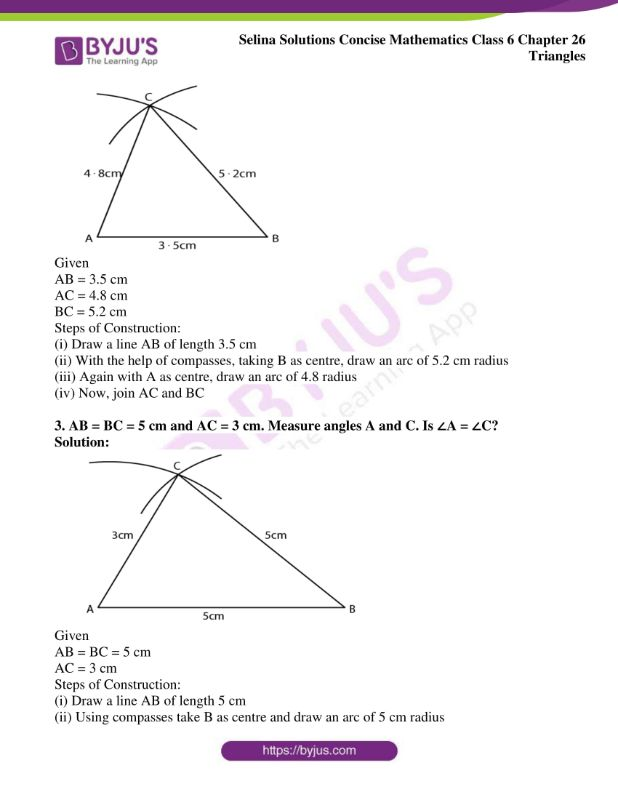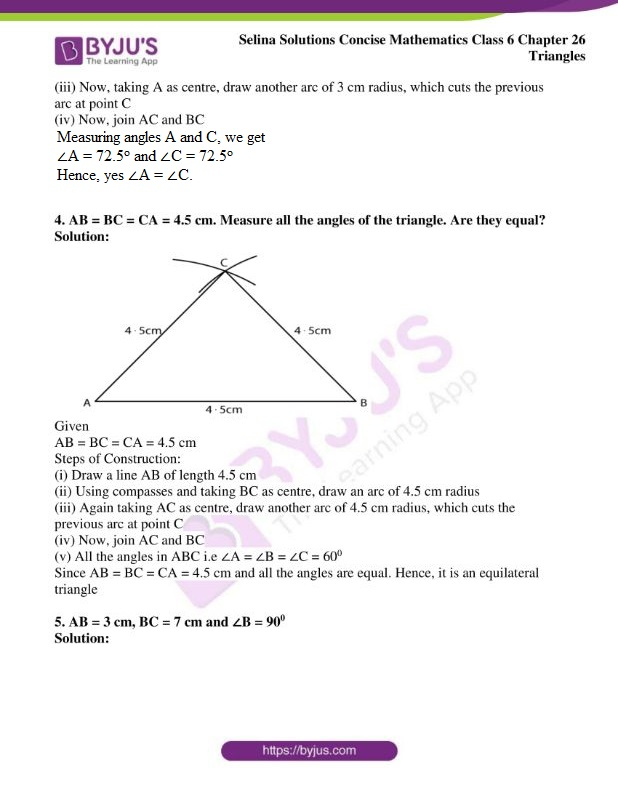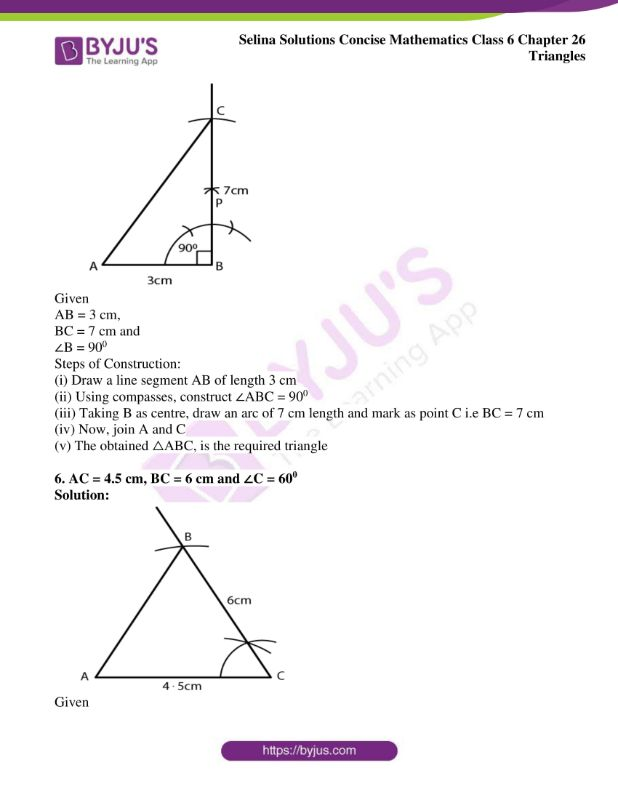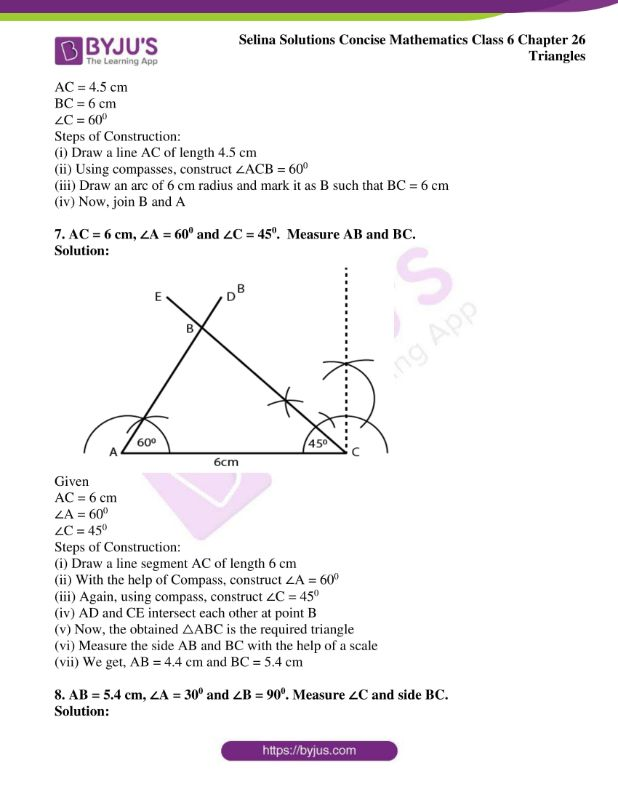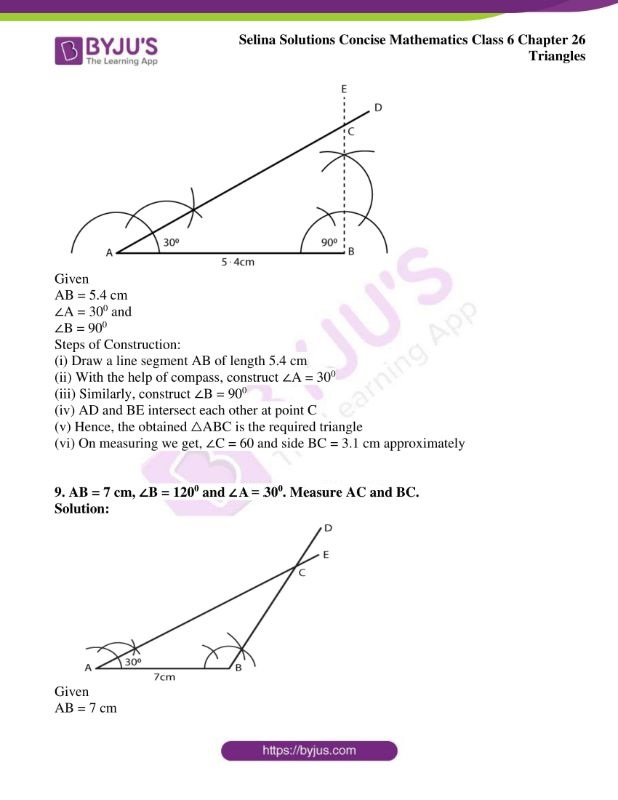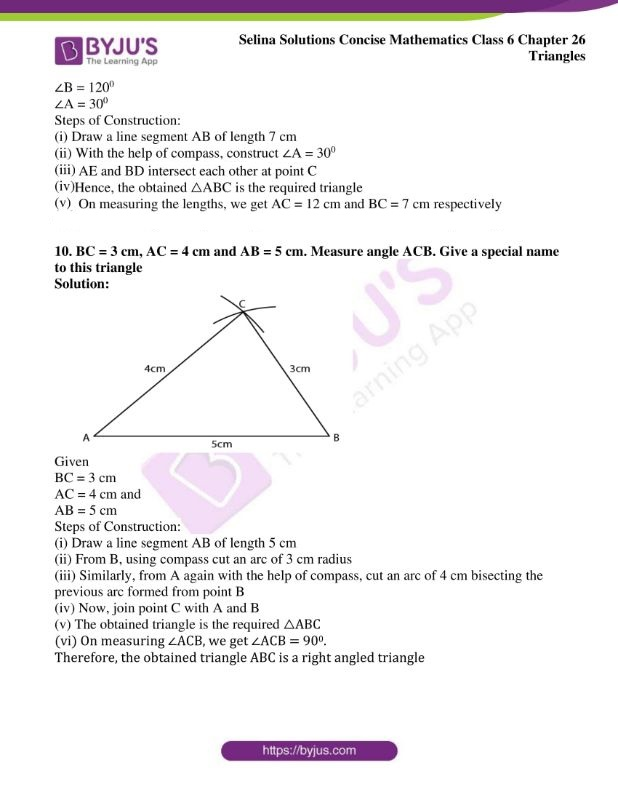### Access another exercise of Selina Solutions Concise Mathematics Class 6 Chapter 26: Triangles

Exercise 26(A) Solutions

### Access Selina Solutions Concise Mathematics Class 6 Chapter 26: Triangles Exercise 26(B)

Exercise 26(B)

1. Construct triangle ABC, when:

AB = 6 cm, BC = 8 cm and AC = 4 cm

Solution: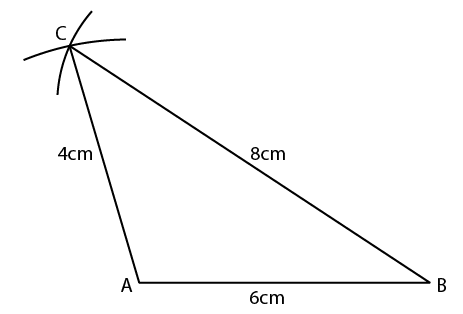Given

AB = 6 cm

BC = 8 cm

AC = 4 cm

Now,

Steps of Construction:

(i) Draw a line AB of length 6 cm

(ii) Using compasses, take B as centre, and draw an arc of 8 cm radius

(iii) Again, taking A as centre, draw another arc of 4 cm radius, which cuts the previous arc at point C

(iv) Now join AC and BC

The obtained triangle ABC is the required triangle.

2. AB = 3.5 cm, AC = 4.8 cm and BC = 5.2 cm

Solution: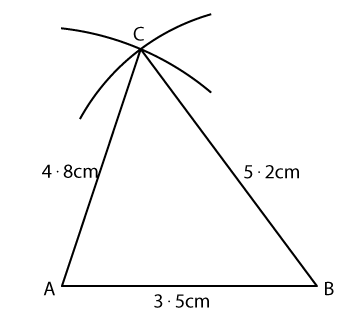Given

AB = 3.5 cm

AC = 4.8 cm

BC = 5.2 cm

Steps of Construction:

(i) Draw a line AB of length 3.5 cm

(ii) With the help of compasses, taking B as centre, draw an arc of 5.2 cm radius

(iii) Again with A as centre, draw an arc of 4.8 radius

(iv) Now, join AC and BC

3. AB = BC = 5 cm and AC = 3 cm. Measure angles A and C. Is ∠A = ∠C?

Solution: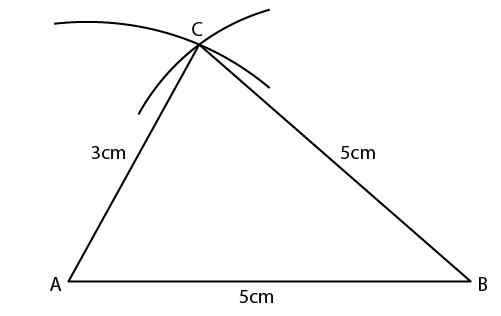Given

AB = BC = 5 cm

AC = 3 cm

Steps of Construction:

(i) Draw a line AB of length 5 cm

(ii) Using compasses take B as centre and draw an arc of 5 cm radius

(iii) Now, taking A as centre, draw another arc of 3 cm radius, which cuts the previous arc at point C

(iv) Now, join AC and BC

Measuring angles A and C, we get
∠A = 72.5o and ∠C = 72.5o
Hence, yes ∠A = ∠C.

4. AB = BC = CA = 4.5 cm. Measure all the angles of the triangle. Are they equal?

Solution: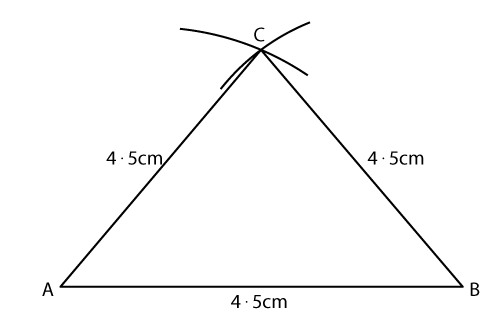Given

AB = BC = CA = 4.5 cm

Steps of Construction:

(i) Draw a line AB of length 4.5 cm

(ii) Using compasses and taking BC as centre, draw an arc of 4.5 cm radius

(iii) Again taking AC as centre, draw another arc of 4.5 cm radius, which cuts the previous arc at point C

(iv) Now, join AC and BC

(v) All the angles in ABC i.e ∠A = ∠B = ∠C = 600

Since AB = BC = CA = 4.5 cm and all the angles are equal. Hence, it is an equilateral triangle

5. AB = 3 cm, BC = 7 cm and ∠B = 900

Solution: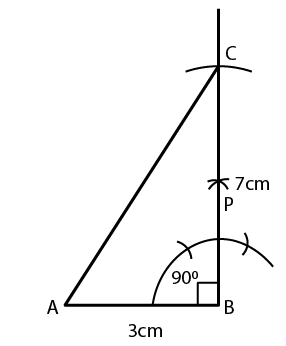Given

AB = 3 cm,

BC = 7 cm and

∠B = 900

Steps of Construction:

(i) Draw a line segment AB of length 3 cm

(ii) Using compasses, construct ∠ABC = 900

(iii) Taking B as centre, draw an arc of 7 cm length and mark as point C i.e BC = 7 cm

(iv) Now, join A and C

(v) The obtained △ABC, is the required triangle

6. AC = 4.5 cm, BC = 6 cm and ∠C = 600

Solution: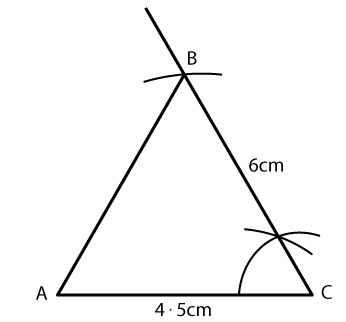Given

AC = 4.5 cm

BC = 6 cm

∠C = 600

Steps of Construction:

(i) Draw a line AC of length 4.5 cm

(ii) Using compasses, construct ∠ACB = 600

(iii) Draw an arc of 6 cm radius and mark it as B such that BC = 6 cm

(iv) Now, join B and A

7. AC = 6 cm, ∠A = 600 and ∠C = 450. Measure AB and BC.

Solution: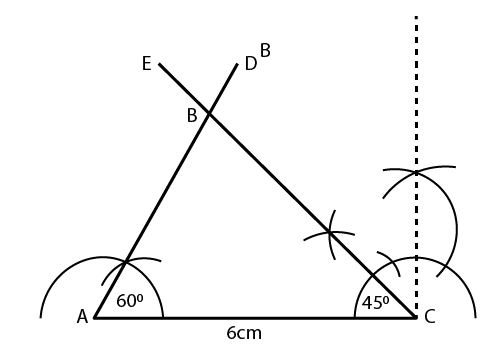Given

AC = 6 cm

∠A = 600

∠C = 450

Steps of Construction:

(i) Draw a line segment AC of length 6 cm

(ii) With the help of Compass, construct ∠A = 600

(iii) Again, using compass, construct ∠C = 450

(iv) AD and CE intersect each other at point B

(v) Now, the obtained △ABC is the required triangle

(vi) Measure the side AB and BC with the help of a scale

(vii) We get, AB = 4.4 cm and BC = 5.4 cm

8. AB = 5.4 cm, ∠A = 300 and ∠B = 900. Measure ∠C and side BC.

Solution: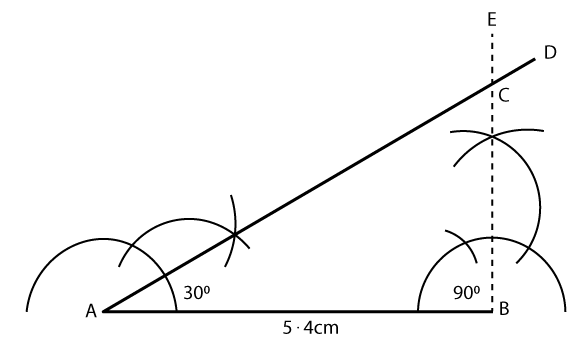Given

AB = 5.4 cm

∠A = 300 and

∠B = 900

Steps of Construction:

(i) Draw a line segment AB of length 5.4 cm

(ii) With the help of compass, construct ∠A = 300

(iii) Similarly, construct ∠B = 900

(iv) AD and BE intersect each other at point C

(v) Hence, the obtained △ABC is the required triangle

(vi) On measuring we get, ∠C = 60 and side BC = 3.1 cm approximately

9. AB = 7 cm, ∠B = 1200 and ∠A = 300. Measure AC and BC.

Solution: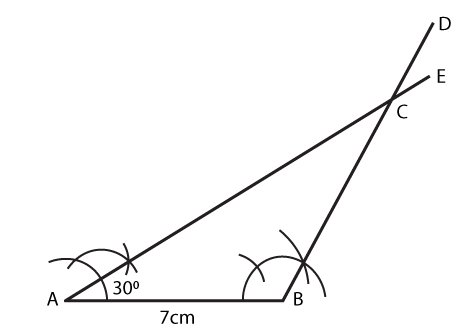Given

AB = 7 cm

∠B = 1200

∠A = 300

Steps of Construction:

(i) Draw a line segment AB of length 7 cm

(ii) With the help of a compass, construct ∠A = 300

(iii) AE and BD intersect each other at point C

(iv) Hence, the obtained △ABC is the required triangle

(v) On measuring the lengths, we get AC = 12 cm and BC = 7 cm respectively

10. BC = 3 cm, AC = 4 cm and AB = 5 cm. Measure angle ACB. Give a special name to this triangle

Solution: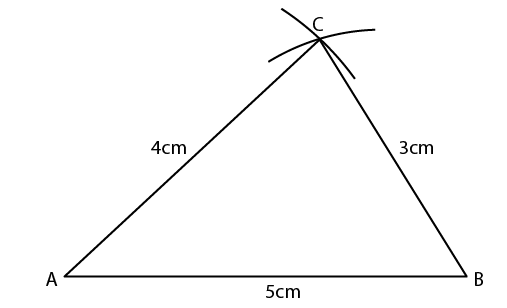Given

BC = 3 cm

AC = 4 cm and

AB = 5 cm

Steps of Construction:

(i) Draw a line segment AB of length 5 cm

(ii) From B, using compass cut an arc of 3 cm radius

(iii) Similarly, from A again with the help of compass, cut an arc of 4 cm bisecting the previous arc formed from point B

(iv) Now, join point C with A and B

(v) The obtained triangle is the required △ABC

(vi) On measuring ∠ACB, we get ∠ACB = 900.

Therefore, the obtained triangle ABC is a right angled triangle